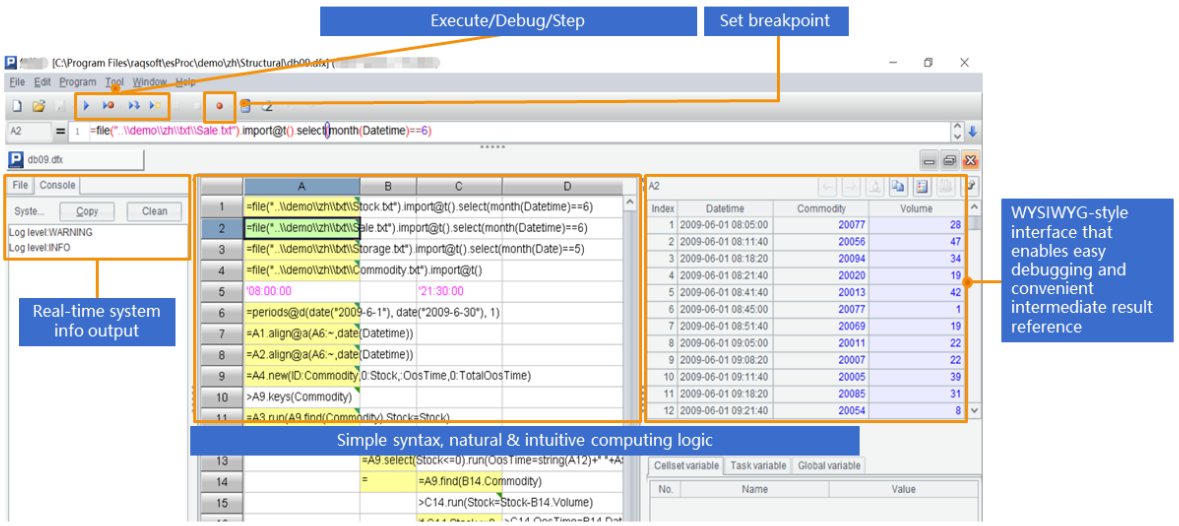# Java 有什么开源包能做类似 SQL 运算的？

SPL实现这些结构化数据处理很方便，比如读取Orders.txt的全部记录：

```…
Class.forName("com.esproc.jdbc.InternalDriver");
Connection connection   =DriverManager.getConnection("jdbc:esproc:local://");
Statement statement =   connection.createStatement();

String str="=T(\"D:/data/Orders.txt\")";
ResultSet result =   statement.executeQuery(str);
…
```

```//条件查询
str="=T(\"D:/data/Orders.txt\").select(Amount>1000   && Amount<=3000 && like(Client,\"*S*\"))";
//排序
str ="=T(\"D:/data/Orders.txt\").sort(Client,-Amount)";
//分组汇总
str ="=T(\"D:/data/Orders.txt\").groups(year(OrderDate);sum(Amount))";
//关联
str ="=join(T (\"D:/data/Orders.txt\"):O,SellerId;   T(\"D:/data/Employees.txt\"):E,EId).new(O.OrderID,O.Client,O.SellerId,O.Amount,O.OrderDate,   E.Name,E.Gender,E.Dept)";```

`str="\$SELECT    year(OrderDate),sum(Amount) from Orders.txt group by year(OrderDate)  "`

SPL脚本可以像上面那样内嵌于JAVA代码（类似SQL）；遇到步骤较多，可能频繁修改，需要降低耦合性的运算，SPL脚本也可以外置于脚本文件（类似存储过程）。比如：找出销售额累计占到一半的前n个大客户，并按销售额从大到小排序。先将SPL脚本存为文件：

 A B 1 = T("D:/data/sales.csv").sort(amount:-1) 取数并逆序排序 2 =A1.cumulate(amount) 计算累计序列 3 =A2.m(-1)/2 最后的累计值即是总和 4 =A2.pselect(~>=A3) 超过一半的位置 5 =A1(to(A4)) 按位置取值

``` …
Class.forName("com.esproc.jdbc.InternalDriver");
Connection connection   =DriverManager.getConnection("jdbc:esproc:local://");
Statement statement =   connection.createStatement();
ResultSet result =   statement.executeQuery("call bigCustomer ()");
...```

 A B 1 =T("d:/AAPL.xlsx") 读Excel文件，首行为列名 2 =a=0,A1.max(a=if(price>price[-1],a+1,0)) 求最长连续上涨天数